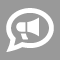#Blogs

## GRE Math Blogs

Summary: Alas, to get that perfect score, you have to reconsider everything you have been taught at school.   My system of preparation for the standardized tests in mathematics (ACT, SAT, SAT2, GRE, etc.) is somewhat unique and unconventional. In fact, it goes against the grain of most of what you have been taught in school... and likely even in a test-prep class, if you have taken one. Sound a bit unnerving? Perhaps. But consider this: those same math teachers who tell you what to do, had most certainly not scored well themselves when they were your age. What is more, chances are good that they cannot score that well now, either. That's because their ways are... well, unduly complicated. What is a better approach? First of all, I will teach you how to solve 95% of all questions mentally, without writing a thing. Why bother, you may ask. Several reasons. One, it will teach you—anew—what you once knew but have since forgotten: the mathematical imagination... read more

Standardized test math doesn't behave like normal math. On a normal math test, your knowledge of the concepts and material is being tested, using (hopefully) fair test questions. On a standardized test, though, they're looking for you to think outside the box, to apply math concepts and algorithms to unusual situations, and to really understand what they're looking for and find the quickest way to go about it. Let's take a question from a recent GRE student's lesson: If 4x – 5y = 10 and 6y – 3x = 22, then what is x + y? Now, this is a set of two equations with two variables each, so it looks to me like a perfect candidate for solving as a system. If I were solving this one on a regular math test, I'd start off trying the substitution method, since I'm more comfortable with that one. So let's explore that one first: I'll start by solving the first equation for y: 4x – 5y = 10 - 5y = 10 – 4x y = (-10/5) – (4/-5)x y = -2 + (4/5)x Then... read more